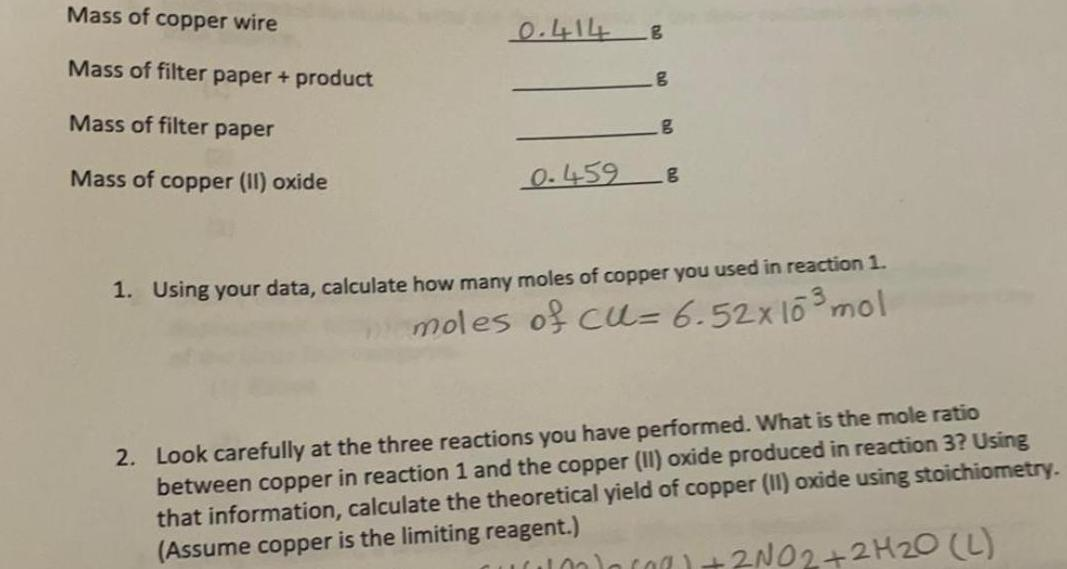Question:

# Mass of copper wire Mass of filter paper + product Mass of

Last updated: 8/5/2022Mass of copper wire Mass of filter paper + product Mass of filter paper Mass of copper (II) oxide 1. Using your data, calculate how many moles of copper you used in reaction 2. Look carefully at the three reactions you have performed. What is the mole ratio between copper in reaction 1 and the copper (II) oxide produced in reaction 3? Using that information, calculate the theoretical yield of copper (II) oxide using stoichiometry. (Assume copper is the limiting reagent.)